### IMO Shortlist 1987 problem 20

Kvaliteta:
Avg: 0,0
Težina:
Avg: 0,0
Let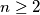$n\ge2$ be an integer. Prove that if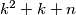$k^2+k+n$ is prime for all integers$k$ such that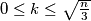$0\le k\le\sqrt{n\over3}$, then$k^2+k+n$ is prime for all integers$k$ such that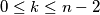$0\le k\le n-2$.(IMO Problem 6)

Original Formulation

Let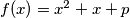$f(x) = x^2 + x + p$,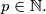$p \in \mathbb N.$ Prove that if the numbers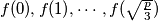$f(0), f(1), \cdots , f(\sqrt{p\over 3} )$ are primes, then all the numbers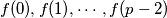$f(0), f(1), \cdots , f(p - 2)$ are primes.

Proposed by Soviet Union.
Izvor: Međunarodna matematička olimpijada, shortlist 1987